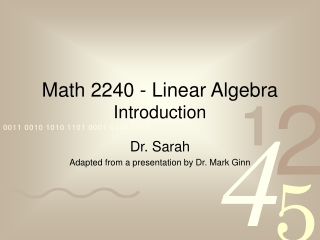DownloadDownload PresentationMath 2240 - Linear Algebra Introduction

# Math 2240 - Linear Algebra Introduction

Télécharger la présentation## Math 2240 - Linear Algebra Introduction

- - - - - - - - - - - - - - - - - - - - - - - - - - - E N D - - - - - - - - - - - - - - - - - - - - - - - - - - -
##### Presentation Transcript

1. Math 2240 - Linear AlgebraIntroduction Dr. Sarah Adapted from a presentation by Dr. Mark Ginn

2. What is Linear Algebra? • develops from the idea of trying to solve and analyze systems of linear equations • linear algebra makes it possible to work with large arrays of data (web pictures…) • has many applications in many diverse fields. (computer graphics, chemistry, biology, differential equations, economics, business, psychology, engineering,...)

3. Great area in which to use technology (Maple) since real life problems often involve computations which are easily done on a computer, but would take a long time to do by hand. • Some of you might want to just use the computer, and skip the theory and proofs, but if we don’t understand the theory, then it can be very hard to appreciate and interpret computer results.

4. What is a linear equation? • A linear equation is an equation of the form, anxn+ an-1xn-1+ . . . + a1x1 = b. • A solution to a linear equation is an assignment of values to the variables (xi’s) that make the equation true.

5. What is a system of linear equations? • A system of linear equations is simply a set of linear equations. i.e. a1,1x1+ a1,2x2+ . . . + a1,nxn = b1 a2,1x1+ a2,2x2+ . . . + a2,nxn = b2 . . . am,1x1+ am,2x2+ . . . + am,nxn = bm

6. A solution to a system of equations is simply an assignment of values to the variables that satisfies (is a solution to) all of the equations in the system. • If a system of equations has at least one solution, we say it is consistent. • If a system does not have any solutions we say that it is inconsistent.

7. Examples This is a consistent system as x =1, y =1 is a solution. This is an inconsistent system. Why??

8. How many solutions does this system have? Let’s look at this graphically in Maple.

9. How many solutions could a system have? • As we saw, a system of linear equations in two variables can have no solutions, one solution, or infinitely many solutions. Any other possibilities? Why? Later in the course, we’ll prove this is also true for any number of variables. • We also saw that graphing is one way to find the solutions. But, visualization is hard in more than 3 dimensions.

10. Solving systems algebraically • Consider the following system, What are its solution(s)?

11. We say that a system in this form is in row -echelon form. We’ll learn more about what this means in the next section. • We can find the solutions to a system in row-echelon form using back substitution. x=1,y=2,z=3

12. Solving a system not in r-e form. • We say that two systems of equations (or equations) are equivalent, if they have exactly the same solutions. • One method of solving a system of equations is to transform it to an equivalent system in r-e form, which we can then solve via back substitution.

13. Operations that lead to equivalent systems 1. Interchange two equations. 2. Multiply an equation by a nonzero constant. 3. Add a multiple of an equation to another equation.

14. Using these three operations we can solve a system of linear equations in the following manner. 1. Save the x term in the first equation and use it to eliminate all other x terms. 2. Ignore the first equation and use the second term in the second equation to eliminate all other second terms in the remaining equations below it. 3. Continue in this manner until the system is in r-e form.

15. Example on using this technique to solve a system of equations and then write the infinite solutions in parametric form (p. 12 #41).